• 目标空间椭圆曲线： ⎧⎩⎨⎪⎪⎪⎪⎪⎪⎪⎪⎪⎪⎪⎪x(t)=y(t)=z(t)=1425(−177t∓219−−√875−3t(27t+50)−−−−−−−−−−−−−−−√+450)1425(159t±919−−√875−3t(27t+50)−−−−−−−−−−−−−...
目标

空间椭圆曲线： ⎧⎩⎨⎪⎪⎪⎪⎪⎪⎪⎪⎪⎪⎪⎪x(t)=y(t)=z(t)=1425(−177t∓219−−√875−3t(27t+50)−−−−−−−−−−−−−−−√+450)1425(159t±919−−√875−3t(27t+50)−−−−−−−−−−−−−−−√+100)t<!--//--><![CDATA[//><!--
\left\{\quad
\begin{array}{cl}
x(t)=&\!\!\!\!\!\frac{1}{425} \left(-177 t \mp 2 \sqrt{19} \sqrt{875-3 t (27 t+50)}+450\right) \\
y(t)= &\!\!\!\!\!\frac{1}{425} \left(159 t \pm 9 \sqrt{19} \sqrt{875-3 t (27 t+50)}+100\right) \\
z(t)=&\qquad\qquad t \\
\end{array}
\right.
//--><!]]>ParametricPlot3D[{{1/425 (450-177z-2Sqrt[19(875-81z^2-150z)]),1/425 (159z+100+9Sqrt[19(875-81z^2-150z)]),z},{1/425 (450-177z+2Sqrt[19(875-81z^2-150z)]),1/425 (159z+100-9Sqrt[19(875-81z^2-150z)]),z}},{z,-30,30},PlotStyle->{Directive[Orange,Opacity,Specularity[White,30]],Directive[Orange,Opacity,Specularity[White,30]]},ImageSize->700,PlotRangePadding->Scaled[.1],PlotPoints->1500,AxesLabel->{Style["x",Black,18,FontFamily->"Times New Roman",Italic,Bold],Style["y",Black,18,FontFamily->"Times New Roman",Italic,Bold],Style["z",Black,18,FontFamily->"Times New Roman",Italic,Bold]}]/.Line[pts_,rests___]:>Tube[pts,0.06,rests]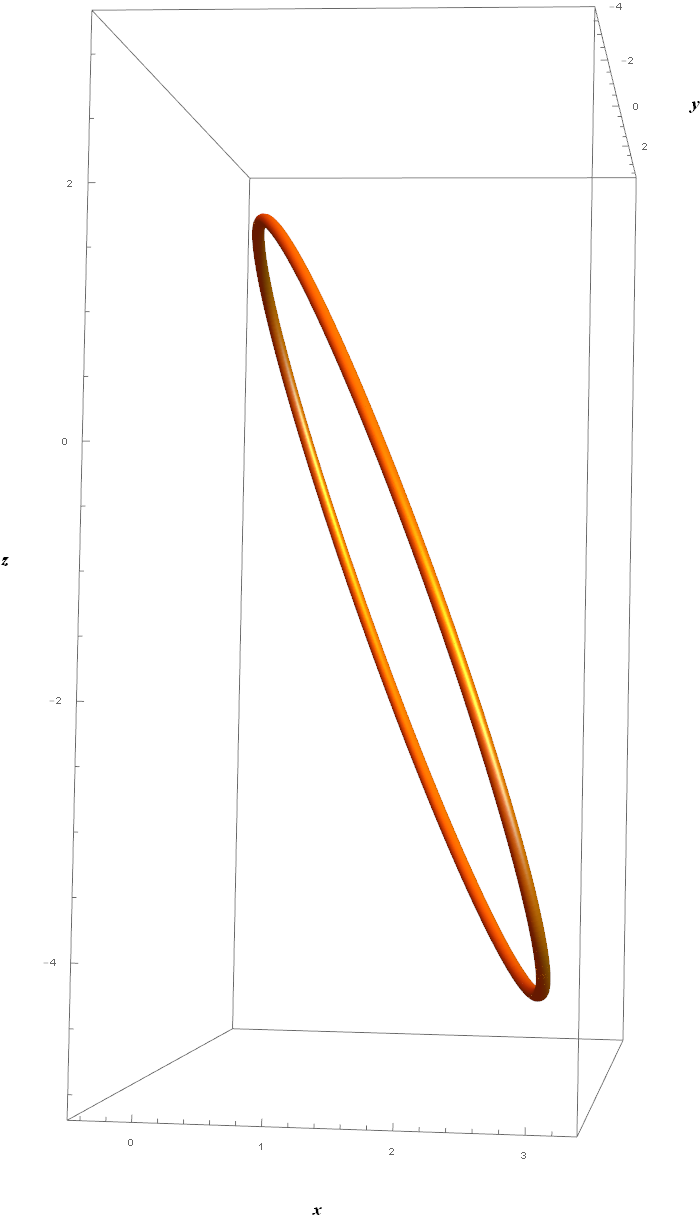经正交变换到坐标平面上的任意隐函数方程。

求解

这个椭圆在平面 π:9X+2Y+3Z−10=0<!--//--><![CDATA[//><!--
\pi:{9 X+ 2 Y+ 3Z -10=0}
//--><!]]> 上。简单的思路是，先把它正投影到该平面，然后再把该平面反射到某个坐标平面上，比如 xoy<!--//--><![CDATA[//><!--
xoy
//--><!]]>。

大致的步骤是：

求π<!--//--><![CDATA[//><!--
\pi
//--><!]]> 上的正投影矩阵（齐次坐标表示），计算出投影之后……参数方程的形式没有发生任何变化；
求 π<!--//--><![CDATA[//><!--
\pi
//--><!]]> 到 xoy<!--//--><![CDATA[//><!--
xoy
//--><!]]> 的相互反射矩阵，利用齐次坐标和Householder矩阵表示法，这个也很容易得到，从而得到xoy<!--//--><![CDATA[//><!--
xoy
//--><!]]> 坐标平面上的参数方程，坐标的 z<!--//--><![CDATA[//><!--
z
//--><!]]> 项必然是 0<!--//--><![CDATA[//><!--
0
//--><!]]>, 否则就是你算错了；
利用 Eliminate 或 GroebnerBasic 方法，对 x(t)，y(t)<!--//--><![CDATA[//><!--
x(t)，y(t)
//--><!]]> 进行消元，消去参数就得到了 F(x,y)=0<!--//--><![CDATA[//><!--
F(x,y)=0
//--><!]]>  形式的隐函数方程；但是可能是关于 x,y<!--//--><![CDATA[//><!--
x,y
//--><!]]>的 四次的多项式形式。它相当于求解的时候无法排除的另外一个椭圆被引入，去掉即可。
用Reduce的方法化简，或者干脆对 x<!--//--><![CDATA[//><!--
x
//--><!]]> (或 y<!--//--><![CDATA[//><!--
y
//--><!]]> )求一元四次方程的符号解，xi(y)=fi(y),i=1,2,3,4<!--//--><![CDATA[//><!--
x_i(y)=f_i(y),\quad i=1,2,3,4
//--><!]]>。每个符号解的表达式自然对应于一段椭圆弧曲线的隐函数方程。把两个有效的隐函数形式的表达式作为因子相乘，得到的就是拼接起来的所求的椭圆的隐函数方程。
ContourPlot[{x==1/1374106940 (1764833994+116325489 Sqrt+383223186 y-56903469 Sqrt y-7 \[Sqrt](370882257712465226+21496012552753644 Sqrt-2437721560491300 y+734799305617824 Sqrt y-39873980011335030 y^2-2840782215729132 Sqrt y^2)),x==1/1374106940 (1764833994+116325489 Sqrt+383223186 y-56903469 Sqrt y+7 \[Sqrt](370882257712465226+21496012552753644 Sqrt-2437721560491300 y+734799305617824 Sqrt y-39873980011335030 y^2-2840782215729132 Sqrt y^2)),x==1/16165964 (20038590+1467345 Sqrt-1024722 y-792645 Sqrt y-5 Sqrt[108441336840026+2474011696404 Sqrt-1949543609220 y+311578887504 Sqrt y-10910839196070 y^2-464210336772 Sqrt y^2]),x==1/16165964 (20038590+1467345 Sqrt-1024722 y-792645 Sqrt y+5 Sqrt[108441336840026+2474011696404 Sqrt-1949543609220 y+311578887504 Sqrt y-10910839196070 y^2-464210336772 Sqrt y^2])},{x,-3,7},{y,-5,5},PlotPoints->300,ImageSize->600,ContourStyle->{Red,Blue,Magenta,Green}]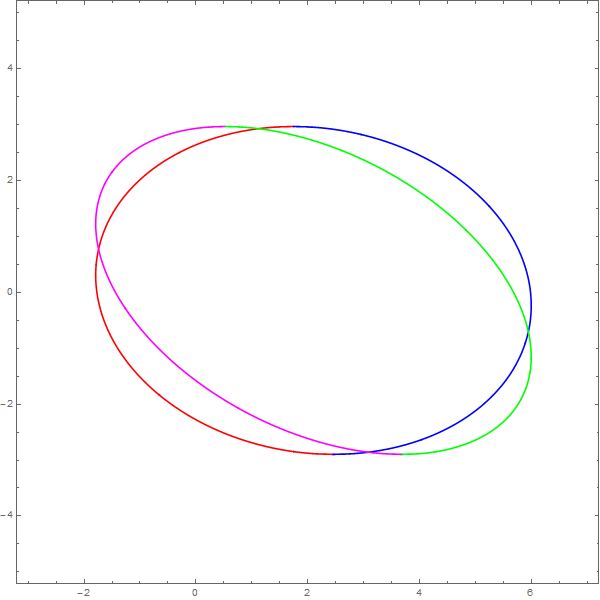最后求得的隐函数方程是：

8082982x2+3x((341574+26421594−−√)y−55(121446+889394−−√))<!--//--><![CDATA[//><!--
8082982 x^2+3 x \left(\left(341574+264215 \sqrt{94}\right) y-55 \left(121446+8893 \sqrt{94}\right)\right)
//--><!]]>+9(1143967+4546594−−√)y2−60(108762+2194194−−√)y−9412594−−√−65170775=0<!--//--><![CDATA[//><!--
+9 \left(1143967+45465 \sqrt{94}\right)
y^2-60 \left(108762+21941 \sqrt{94}\right) y-94125 \sqrt{94}-65170775=0
//--><!]]>画出来是这样的：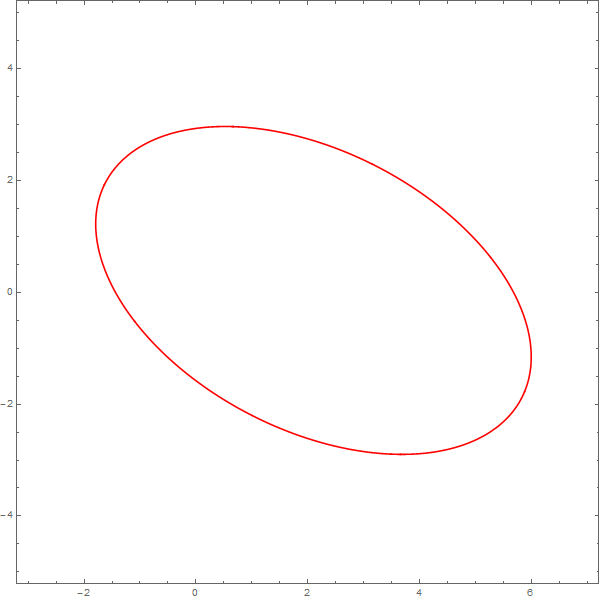展开全文函数
• 在构造适用于双线性对的椭圆曲线的方法中,通常将椭圆曲线的参数表示成有理多项式,为有效地生成椭圆曲线,要求复乘方程的次数应小于3。通过将椭圆曲线参数看作数域元素,提出了一种构造合适的有理多项式的方法,使得复乘...
• 有限域椭圆曲线点的阶 ...考虑K=kG ，其中K、G为椭圆曲线Ep(a,b)上的点，其中a,b是二元三次椭圆方程的参数，n为G的阶（nG=O∞ ），k为小于n的整数。则给定k和G，根据加法法则，计算K很容易但反过来，给
有限域椭圆曲线点的阶

如果椭圆曲线上一点P，存在最小的正整数n使得数乘nP=O∞ ,则将n称为P的阶
若n不存在，则P是无限阶的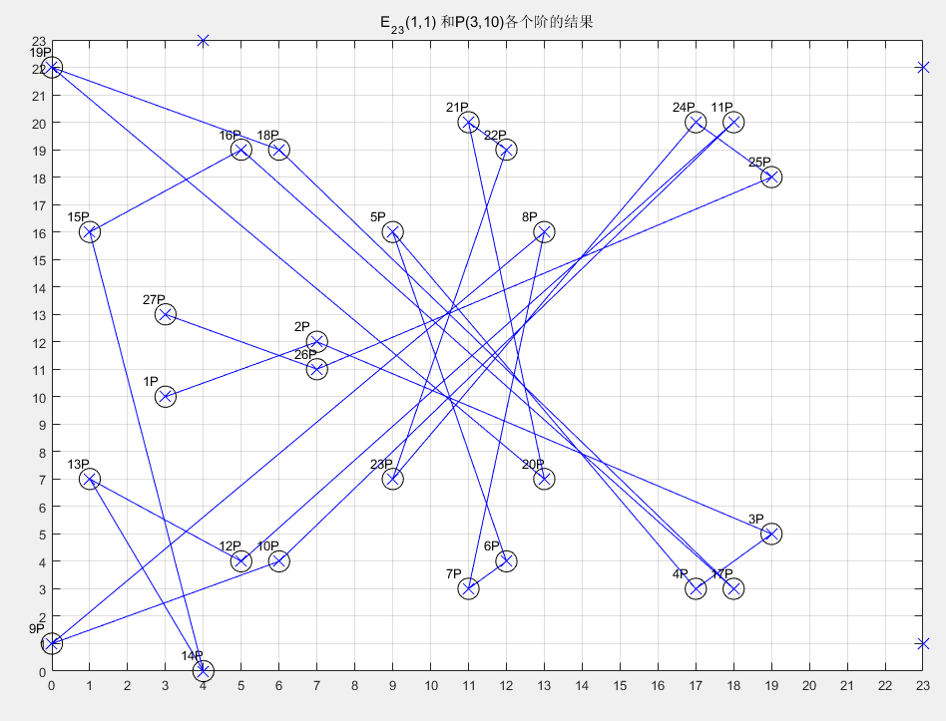计算可得27P=-P=(3,13)

所以28P=O ∞ P的阶为28

这些点做成了一个循环阿贝尔群，其中生成元为P，阶数为29。显然点的分布与顺序都是杂乱无章

椭圆曲线加密

考虑K=kG ，其中K、G为椭圆曲线Ep(a,b)上的点，其中a,b是二元三次椭圆方程的参数，n为G的阶（nG=O∞ ），k为小于n的整数。则给定k和G，根据加法法则，计算K很容易但反过来，给定K和G，求k就非常困难。因为实际使用中的ECC原则上把p取得相当大，n也相当大，要把n个解点逐一算出来列成上表是不可能的。这就是椭圆曲线加密算法的数学依据

点G称为基点（base point）

k（k<n）为私有密钥（privte key）

K为公开密钥（public key)

展开全文• 前面已经说了，素域Fp上的椭圆曲线E由（仿射）方程y2＝x3＋ax＋b决定，所以Jacobi射影点P＝(X,Y,Z)在不在曲线上就看这个点是不是满足射影方程y2＝x3＋axz4＋bz6。带入P点分别计算方程左右两边再做比较即可。 同样地...
判断一个点在曲线上的理论依据是什么呢？前面已经说了，素域Fp上的椭圆曲线E由（仿射）方程y2＝x3＋ax＋b决定，所以Jacobi射影点P＝(X,Y,Z)在不在曲线上就看这个点是不是满足射影方程y2＝x3＋axz4＋bz6。带入P点分别计算方程左右两边再做比较即可。

同样地，如果P点的Z分量为1，计算量就可以大大降低，这就是在点的定义中加入参数Z_is_one的好处。

下面来解释判断点是否在曲线上的函数。

───────────────────────────────────────

int EC_POINT_is_on_curve(const EC_GROUP *group, const EC_POINT *point)

功能：    判断点是否在曲线上

输入：    group，point

输出：    －

返回：    1【在曲线上】 or 0【不在曲线上】 or －1【出错】

出处：    ec_lib.c

调用：     ▼   int ec_GFp_simple_is_on_curve(const EC_GROUP *group, const EC_POINT *point)

───────────────────────────────────────

展开全文openssl
• 先把标题放在这里,内容我慢慢准备,一点点添加1. 一个简单例子从最简单情况出发,连续可微封闭曲线为边界,最简单例子是”圆”....所以我选择了如下参数方程的椭圆： {x=y=5costcosθ−3sints
先把标题放在这里,内容我慢慢准备,一点点添加1.

一个简单的例子

从最简单的情况出发,连续可微的封闭曲线为边界的,最简单例子是”圆”.
——“什么？你忽悠我？！”
为了不至于激起不满，我临时决定把这个简单的例子更改为椭圆。当然，如果椭圆的长短轴跟直角坐标系的坐标轴平行，其实也还简单，所以让它再旋转一个角度θ<!--//--><![CDATA[//><!--
\theta
//--><!]]>。所以我选择了如下参数方程的椭圆： {x=y=5costcosθ−3sintsinθ3cosθsint+5costsinθ<!--//--><![CDATA[//><!--
\left\{\;
\begin{array}{rl}
x=&5 \cos t \cos \theta -3 \sin t \sin \theta  \\
y=&3 \cos \theta  \sin t+5 \cos t \sin \theta \\
\end{array}
\right.
//--><!]]>为了把曲线画出来, 让θ=30o<!--//--><![CDATA[//><!--
\theta=30^o
//--><!]]>, 从而其参数方程变为: ⎧⎩⎨⎪⎪⎪⎪x=y=12(53√cost−3sint)12(5cost+33√sint)<!--//--><![CDATA[//><!--
\left\{\;
\begin{array}{rl}
x=&\frac{1}{2} \left(5 \sqrt{3} \cos t-3 \sin t\right) \\
y=&\frac{1}{2} \left(5 \cos t+3 \sqrt{3} \sin t\right) \\
\end{array}
\right.
//--><!]]>ParametricPlot[RotationTransform[Pi/6][curve], {t, 0, 2 Pi}] /.  Line[x_] :> {Red, Line[x]}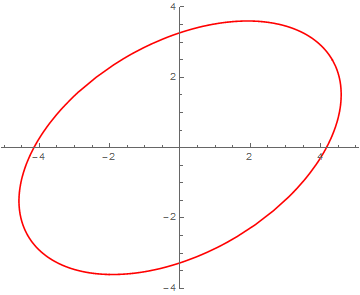要判断任给点P=(x0,y0)<!--//--><![CDATA[//><!--
P=(x_0,y_0)
//--><!]]>是不是在图中的浅蓝色区域: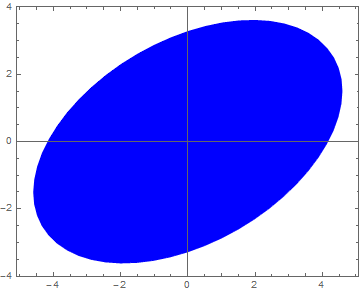不知道常用的都是什么办法? 2.
.
至少对圆的情形,判断的方法是到圆心的距离跟圆的半径作比较. 但是椭圆不同了,而且旋转了θ<!--//--><![CDATA[//><!--
\theta
//--><!]]>角,让自然参数t<!--//--><![CDATA[//><!--
t
//--><!]]>也不直接对应于P<!--//--><![CDATA[//><!--
P
//--><!]]>点的方位了,只是稍微增加了一点点麻烦而已,仍然可以找出椭圆上的边界点,然后对比到椭圆圆心的距离. 这个方法太原始了,而且不易推广,显然不是我想说的.

看看math.stackexchange.com上人家怎么说吧.如何确定任一点是否在二次曲线内,我当然不是为了转一个链接而卖关子. 链接里的方法主要还是对方程为隐函数g(x,y)=Ax2+Bxy+Cy2+Dx+Ey+F=0<!--//--><![CDATA[//><!--
g(x,y) = Ax^2+Bxy+Cy^2+Dx+Ey+F=0
//--><!]]>形式的二次曲线,利用其二次型.但仅适用于二次曲线.

大部分情况下,参数方程更方便,而且两种形式之间互换本来也挺麻烦. 这里介绍基于椭圆的参数方程时,如何判断; 同时,把这个例子里的概念推广到更一般的高于二次的C∞<!--//--><![CDATA[//><!--
C^\infty
//--><!]]>曲线，以及凸的和凹的多边形.

这个概念就是拓扑学中的winding number 或 卷绕数3.

很容易把任意曲线的参数方程: {x=y=x(t)y(t)<!--//--><![CDATA[//><!--
\left\{\;
\begin{array}{rl}
x=& x(t) \\
y=& y(t) \\
\end{array}
\right.
//--><!]]>
改写成极坐标形式: ⎧⎩⎨⎪⎪⎪⎪⎪⎪ρ=θ=x(t)2+y(t)2−−−−−−−−−−√arctan(y(t)x(t)) 特殊的反正切,应该带卦限信息,并兼容x(t)=0<!--//--><![CDATA[//><!--
\left\{\;
\begin{array}{rl}
\rho =& \sqrt{x(t)^2+y(t)^2} \\
\theta=& \arctan\left(\dfrac{y(t)}{x(t)}\right) \text{ 特殊的反正切,应该带卦限信息,并兼容}x(t)=0\\
\end{array}
\right.
//--><!]]>求导数之后(参考维基上的内容winding number 或 卷绕数):

dθ=x(t)y′(t)−y(t)x′(t)x(t)2+y(t)2dt<!--//--><![CDATA[//><!--
d\theta=\dfrac{x(t) y'(t)-y(t) x'(t)}{x(t)^2+y(t)^2}dt
//--><!]]>

曲线x(t)−y(t)<!--//--><![CDATA[//><!--
x(t)-y(t)
//--><!]]>在某点(x0,y0)<!--//--><![CDATA[//><!--
(x_0,y_0)
//--><!]]>的卷绕数定义为: w(x0,y0)=12π∫t1t0(x(t)−x0)y′(t)−(y(t)−y0)x′(t)(x(t)−x0)2+(y(t)−y0)2dt<!--//--><![CDATA[//><!--
\text{w}_{(x_0,y_0)}=\dfrac{1}{2\pi}\int_{t_0}^{t_1}\frac{(x(t)-{x_0}) y'(t)-(y(t)-{y_0})x'(t)}{\left(x(t)-{x_0}\right)^2+\left(y(t)-{y_0}\right)^2}dt
//--><!]]>
对于前面提到的椭圆:t0=0,t1=2π<!--//--><![CDATA[//><!--
t_0=0, t_1=2\pi
//--><!]]>,**当w(x0,y0)<!--//--><![CDATA[//><!--
\rm{w}(x_0,y_0)
//--><!]]>为0时,点在椭圆外,为非0整数时在其内。

这个结论使得用数值积分计算结果作为判据非常合适,因为它对积分的数值计算有很大的允许误差; 从维基还是某本书上提到,winding number在量子力学里面又是一种”量子数”(稍后核实出处)。

用Mathematica代码做个试验:

curve={5Cos[t],3Sin[t]};  (*标准椭圆定义*)
{x[t],y[t]}=RotationTransform[Pi/6]@curve; (*旋转30°的参数形式*)
innerQ[{x0_,y0_}]:=Round[NIntegrate[((x[t]-x0)D[y[t],t]-(y[t]-y0)D[x[t],t])/((x[t]-x0)^2+(y[t]-y0)^2),{t,0,2Pi}]/2/Pi]!=0 (*以卷绕数为判据的函数的定义*)
innerQ /@ {{3, 5}, {3, 2}, {0, 0}, {-4, 1}} (*测试几个点*)
{False, True, True, False}(*输出结果*)

太丑了! 换个表现方式!!

ClearAll["Global*"]
curve={5Cos[t],3Sin[t]};
new=RotationTransform[Pi/6]@curve;
x[t_]:=new[]
y[t_]:=new[]
innerQ[{x0_,y0_}]:=Round[NIntegrate[((x[t]-x0)D[y[t],t]-(y[t]-y0)D[x[t],t])/((x[t]-x0)^2+(y[t]-y0)^2),{t,0,2Pi},AccuracyGoal->6]/2/Pi]!=0
hc=ParametricPlot[u*RotationTransform[Pi/6]@curve,{t,0,2Pi},{u,0,1},PlotRange->{{-5,5},{-4.5,4.5}},PlotPoints->30,MeshFunctions->{#4&},Mesh->{{1.1}},MeshShading->{Yellow,Yellow}]/.Line[_]:>Sequence[];
SeedRandom
(points=RandomReal[{-4,4},{10,2}]);
txt=Text[Style[#,Red,20],points[[#]],{1,0}]&/@Range[Length@points];
v=innerQ/@points;
Join[points,ConstantArray["->",{10,1}],Transpose@{Range},Transpose@{v},2]//MatrixForm
Show[hc,Graphics[{Blue,AbsolutePointSize,Point[#]&/@(1.15points)}],Epilog->{Red,txt},Frame->True,ImageSize->Large]

输出结果是这样滴:

编号
点的坐标
是否在曲线封闭区域之内
1
(-3.99368, -3.47985)
不在
2
(3.91644, 3.75014)
不在
3
(-2.39307, 2.55617)
不在
4
(-3.28189, 3.76561)
不在
5
(-2.16072, 0.90002)
在
6
(-3.22547, 0.39084)
在
7
(-2.93962, -2.14134)
在
8
( 2.20908, 0.40759 )
在
9
(-3.53048, 3.68482)
不在
10
(-3.21401, -3.72518)
不在

……………………………………………………………………………………………………………………………..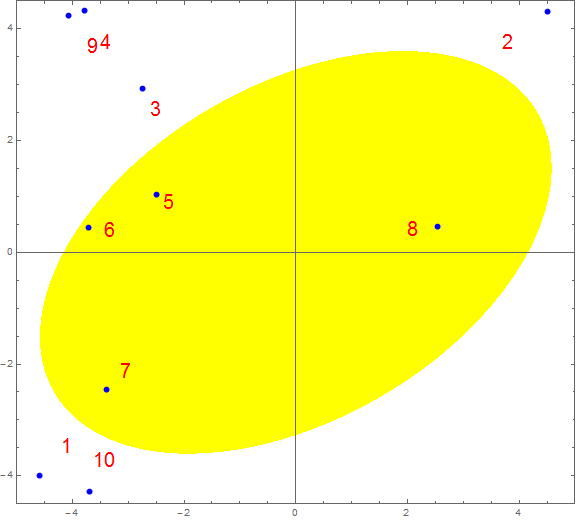卷绕数的几何意义

这个部分希望能够增加一点直观生动的内容。

一个深入点的例子

前面的例子其实不能算简单，我开始后悔没有用”圆“了。

如果说圆和椭圆的情况，都还可以通过测量到”形心距离”之类的方法——即便有些小周折，这让基于winding number 或 卷绕数的方法还是有些不太自在的——”深入点的例子“必须给常规的潜在竞争关系的方法制造足够的麻烦，或者至少要让心目中想要突出的、中意的方法干得更加漂亮！

下面这个例子，一方面仍然保持图形以及参数方程本身在视觉上或表面看上去的足够简单，同时希望让那些初级的方法更加步履维艰，否则我写了这么多不是瞎忙活吗。这条曲线的参数方程是： {x(t)=y(t)=9sin2t+5sin3t9cos2t−5cos3t<!--//--><![CDATA[//><!--
\left\{\quad
\begin{array}{rl}
x(t)=& 9  \sin 2 t+5 \sin 3 t \\
y(t)=& 9 \cos 2 t-5 \cos 3 t \\
\end{array}
\right.
//--><!]]>
把它画出来是这样的，看上去是不是有些似曾相识？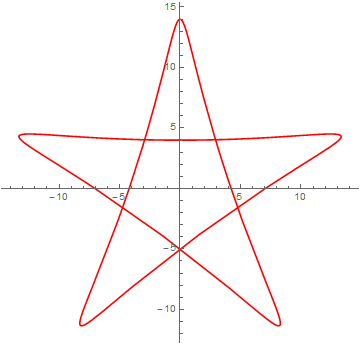在用凹多边形表述发现代码太长之后，我想尝试用曲线近似表达一颗五角星，结果就找到了它。它的边上的肌肤的线条都是C∞<!--//--><![CDATA[//><!--
C^\infty
//--><!]]>光滑的，不像凹多边形那样有棱有角；但是形状有非常近似；所有的边界都可以用一个方程表达，看上去似乎有压缩代码的潜力。如果可以简洁高效地判断出内点外点，只须再作如下填充不就是五角星了吗：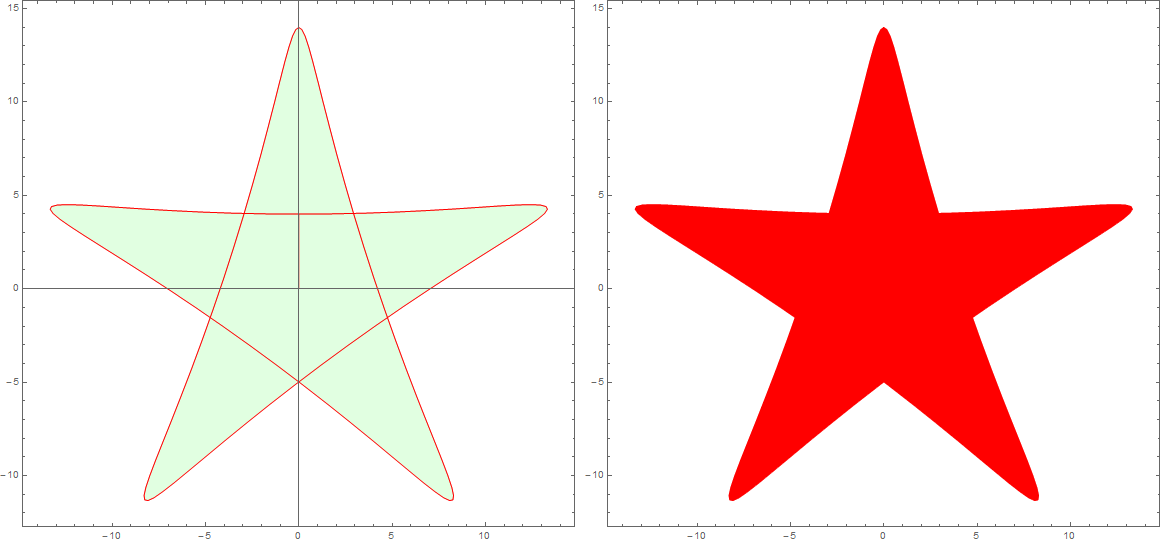代码悄悄地：

Grid@{{ParametricPlot[u {9 Sin[2 t]+5 Sin[3 t],9 Cos[2 t]-5 Cos[3 t]},{t,0,2 Pi},{u,0,1},MeshFunctions->{(*Sqrt@(#1^2+#2^2)*)#4&},Mesh->{{1}},PlotPoints->30,MeshStyle->Red,MeshShading->{{Cyan,LightGreen}},ImageSize->Large]/.Line[x_]:>{Red,Line[x]},ParametricPlot[u {9 Sin[2 t]+5 Sin[3 t],9 Cos[2 t]-5 Cos[3 t]},{t,0,2 Pi},{u,0,1},MeshFunctions->{(*Sqrt@(#1^2+#2^2)*)#4&},Mesh->{{1}},PlotPoints->30,MeshStyle->Red,MeshShading->{{Red,Red}},ImageSize->Large,Axes->False]/.Line[x_]:>{Red,Line[x]}}}

郁闷的是，对它进行判断的方法，跟椭圆的时候没有区别。所以，这部分就不讲了。连个偷着乐的表情都不能发，MarkDown太死板、不灵活。不过算法似乎可以趁机把MarkDown的流程图练习一下, 等着吧。

此外，对于这个例子为何比椭圆情况更进一步没有解释。
对winding number的解释,也缺乏维基那样生动的动态演示.
虽然不是必要的,作为练习还是可以慢慢加上来的.

向多边形的推广

惊艳的其中一部分在这里。 拓扑的确不一样。

卷绕数在五角星绘制中的应用实例

本来问题就是从绘制五星红旗开始的。为了压缩代码，希望找到一个新的判断内外点的算法。现在不论成功与否，贴代码吧。

我只把高级语言当作表达某些意思的工具，但是从来没有去研究关于这类工具的特殊的形式和技巧，无从着手，也不是特别感兴趣。所以，算法在此，进一步压缩只能翘首等别人了。

// NOTE: compile with g++ filename.cpp -std=c++11
#include <iostream>
#include <cmath>
#include <cstdlib>
#include <vector>
//#include <functional>
#define DIM1 600
#define DIM2 400
#define DM1 (DIM1-1)
#define DM2 (DIM2-1)
#define _sq(x) ((x)*(x)) // square
#define _cb(x) abs((x)*(x)*(x)) // absolute value of cube
#define _cr(x) (unsigned char)(pow((x),1.0/3.0)) // cube root

#define pi 3.14159
#define eps 2.02e-16
double arct(double x,double y){//可改写为宏放Rd函数中
return abs(x)>eps?(x>eps?atan(y/x):(y>0?atan(y/x)+pi:atan(y/x)-pi)):(y>0?pi/2:-pi/2);
}

bool inQ(int i,int j,int* px){//改为lambda表达式放GR/BL中
double art,aro;
for(int k=0;k<5;k++){
art[k]=arct((double)(px[k]-i),(double)(px[k+5]-j));//
}
double total=0.;
for(int k=0;k<5;k++){
aro[k]=art[(k+1)%5]-art[k];
while(aro[k]<-pi) aro[k]+=pi;
while(aro[k]>pi) aro[k]-=pi;
total+=aro[k];
}
return abs(total)>0.5;
};//即使修改了也只能作有限压缩，不能达到420字符水平

unsigned char GR(int,int);
unsigned char BL(int,int);

unsigned char RD(int i,int j){
// YOUR CODE HERE
return 255;
}
unsigned char GR(int i,int j){
// YOUR CODE HERE
int p1={100, 135, 43, 157, 65, 40, 149, 81, 81, 149},
p2={184, 220, 183, 207, 205, 168, 181, 191, 161, 199},
p3={183, 208, 204, 185, 220, 50, 22, 59, 27, 42},
p4={221, 259, 229, 239, 252, 135, 133, 157, 120, 156},
p5={220, 254, 237, 231, 258,83, 66, 100, 62, 89};
return inQ(i,j,p1)||inQ(i,j,p2)||inQ(i,j,p3)||inQ(i,j,p4)||inQ(i,j,p5)?255:0;
}
unsigned char BL(int i,int j){
// YOUR CODE HERE
return 0;
}

void pixel_write(int,int);
FILE *fp;
int main(){
fp = fopen("MathPic.ppm","wb");
fprintf(fp, "P6\n%d %d\n255\n", DIM1, DIM2);
for(int j=0;j<DIM2;j++)
for(int i=0;i<DIM1;i++)
pixel_write(i,j);
fclose(fp);
system("pause");
return 0;
}
void pixel_write(int i, int j){
static unsigned char color;
color = RD(i,j)&255;
color = GR(i,j)&255;
color = BL(i,j)&255;
fwrite(color, 1, 3, fp);
}


代码的输出结果ppm经过XnView或Convert转换成png格式之后是这样的：foo bar foo
foo bar foo

Edit

【稍安勿躁，正在加班加点更新】“相濡以沫，不如相忘于江湖。” 我发现庄子就像读过鲁迅的《伤逝》一样，早在几千年前就建议好了该放下的时候要果断放下，可还是有人执迷不悟。——这就是古人的大智慧。“难道我们还不如古人吗？”(后面这个反问句在我高中一同学的作文里常用，每次想起他在班里当众读自己作文里这一句时气势汹汹的样子，我都想回答“是的。在很多方面。”这也是我瞧不起一些文科生和文科公知的原因: 立论完全罔顾事实,单纯依赖辩论技巧. 你他么这样自欺欺人能解决黎曼猜想还是哥德巴赫猜想?) ↩我觉得刚才我写到这里没更新的时候,肯定有点进来的人看了很失望,因为实际上到此为止,除了画了个椭圆,我啥也没讲。会不会觉得博主不太靠谱？那一定是因为你自己更不靠谱！我觉得评价一个人靠谱与否的唯一标准是看此人是不是删过facebook  ↩“拓扑学”听上去挺抽象，但我觉得数学之所以看上去让人望而却步，除了某些基础性的基本功的确需要积累和修炼之外，大部分因为老师的方法有问题。 在深入了解这个概念之前就知道了“判断是内点或外点”时这个概念该怎么用——也就是实际使用的方法是很简单的——，不过，我边写边完善的过程中发现有更多相关而有趣的不同表述，比我原先想象的精彩得多，需要自己细细琢磨，所以更新的速度可能会变慢。学习速度更快的不妨自己先看维基。 ↩`
展开全文微分几何 算法
• 椭圆曲线的方程为或者，选择大整数和参数，其中为素数，或者形为的整数。椭圆群，在中挑选基点，的阶为一个非常大的数，点的阶使得成立的最小正整数。 Alice和Bob之间完成密钥交换的过程如图所示 要破解这种体制...信息安全
• 群建好之后，就可以开始对这个群进行一些基本得操作了，如需要把很多必要信息（如特征p、Weierstrass方程参数a、b、基点和阶等）添加进群里面去，同时在必要时还需要将这些信息读出来。 先来看看对特征p和...openssl
• 为解决该问题,在双曲线形漏斗设计原理基础上,通过推导分析,得出了等比收缩椭圆锥形煤仓漏斗的曲线方程,并确定了漏斗高度、切线角等相关参数,最后给出了使用方法和步骤,对今后同类煤仓设计和施工有较大指导意义...
• P256V1Curve 代表国密SM2推荐的椭圆曲线: 1. 素数域256位椭圆曲线 2. 方程为 Y^2 = X^3 + aX + b 3. 其他参数: p, a, b, n, Gx, Gy 详见国标 4. 在GO语言标准库ellipticCurveParams类基础上增加了参数a...
• Taubin 在文章“由隐式方程定义的平面曲线、曲面和非平面空间曲线的估计，以及边缘和范围图像分割的应用”一文中提出，IEEE Trans。 帕米，卷。 13，第 1115-1138 页，（1991）。 注意：此方法将二次曲线（圆锥...matlab
• 通过研究相关谱曲线族上θ函数可交换性猜想，我们推导了摄动Seiberg-Witten势其他非线性方程，结果证明它具有精确椭圆系统作为通用解。 与WDVV方程相比，新方程获得了非扰动校正，可以直接从可交换性条件...
• 此函数中的参数说明， “mx”是 x 坐标中图案的中心。 “我”是y坐标中图案的中心“a”和“b”是轴。 “sita”是旋转角度（弧度） “f”是图案尺寸常数。 完整的方程是 a((x-mx)*cos(sita)+(y-my)*sin(sita))^2 + b...matlab
• 作出参数曲线的图形： 参数曲线作图的基本格式： plot([x(t),y(t), t=a…b)]); 例1.5.1 作出以下参数方程表示的图形： 这是一个圆心在原点，半径为a的圆。 解 输入以下命令： a:=1.5: plot([a * cos(t), a * sin(t),...
• 首先，包含一组用于二次曲线的函数，用于识别圆锥截面并计算由一般二次方程给出的圆锥截面的显式参数（长半轴、短半轴、旋转矩阵、平移向量）； 或者绘制一个圆锥截面，返回一个 lineseries 对象（对于圆、椭圆和...matlab
• 椭圆标准情况下参数方程用 ( 5 cos(t), 3 sin(t) ) 假设旋转角速度为 \theta, 平移速度为 2\theta (弧度直接变距离) 对参数方程形式向量作旋转和平移,然后对y坐标求极大值可以得到,极大值点对应坐标: 从而...动画 Geogebra
• §1 圆 1. 圆方程 图形 方程 圆心 ...2º 参数方程：   3º 极坐标方程： ρ=R  G( 0, 0)     r=R   1º (x-a)² +(y-b)² =R²解析几何
• 这是一个二维正交椭圆网格（网​​格）生成器，它通过求解Winslow偏微分方程（Elliptic PDE）来工作。 它能够使用拉伸功能和正交性调整算法来修改网格。 该算法通过使用倾斜抛物线切线拟合器计算曲线斜率来工作。 ...
• 此函数从由下式给出的一般圆锥曲线生成点其规范方程的参数 A、B、C、D、E 和 F： A*x^2 + 2*B*x*y + C*y^2 + 2*D*x + 2*E*y + F = 0 点可以吵，噪音的功率可以配置带有噪声功率变量（高斯噪声的标准偏差）。...matlab
• 主要介绍了HTML5 Canvas中绘制椭圆的4种方法,本文讲解了参数方程法、均匀压缩法、三次贝塞尔曲线法、光栅法等4种方法,需要朋友可以参考下
• 椭圆芯手征光纤进行了严格求解,得到了模式场解析解;推出了模式特征方程;并对基模偶模和奇模特征方程进行数值求解;研究了手征参数对不同椭圆比下基模色散曲线、双折射、模间色散等特性影响。
• 层级N空间每个点对应于一维N $$\ mathcal {N}$$ = 4 Landau-Ginzburg理论，它定义在基本单元中具有N个真空度和N个极点的椭圆曲线上。 模曲线Y（N）=ℍ/Γ（N）是NY 1（N）覆盖，并在模型空间中起到谱覆盖...
• 当IPP依赖于一条椭圆曲线（没有其他代数函数）时，可以通过使用零件标识积分，根据椭圆多重对数（eMPL）在算法上求解此类Feynman积分。 然后，我们详细介绍微分方程法。 具体而言，我们表明IPP可以映射到满足a型...
• 我们已经证明，传递矩阵特征值和Bethe方程的基本构造块都可以用椭圆曲线亚纯函数表示。 我们讨论了源自R矩阵第二光谱参数的特定选择潜在自旋一链属性。 我们提供了数值和分析证据，取决于相互作用耦合...
• 高阶椭圆锥齿轮是一种典型非圆锥齿轮传动形式.以空间传动啮合原理为基础,建立了该锥齿轮传动空间球面啮合坐标系.分析了节曲线、齿廓及啮合线之间变化关系;运用空间坐标变换矩阵法创建了该齿轮齿面方程....
• ## 曲线函数

千次阅读 2018-11-29 18:49:36
这些曲线的显示可采用类似于前面讨论的圆和椭圆函数来生成。沿曲线轨迹的位置可直接从表达式y =f (x)或参数方程中得到。此外，还可以使用增量中点算法绘制用隐式函数f(x,y) = 0描述的曲线。 显示一...
• 椭圆与圆不同，不能八分只能四分。...从开始，在方向取单位步长直到区域1和区域2界限处，然后转还为方向单位步长，再覆盖第一象限中剩余的曲线段。不过，每一步都要检测曲线斜率值。 斜率方程： ...python
• 对于这种情况，可将交线的参数方程找到，再用前面的方法作出曲线的图形。 例 3.1.5 作出以下曲线的图形: 解 这是椭圆柱面和旋转抛物面的交线。两曲面的参数方程是： 输入以下命令： with(plots): a:=1.2????️=1: ...
• ## 椭圆拟合算法总结

万次阅读 2015-07-31 15:26:38
椭圆拟合算法 椭圆拟合在医疗图像、工程设计、交通识别、生物识别、人工智能、林火蔓延等领域具有广泛应用，本文总结前人研究综合...关键在于估计的参数单位. p0=[1 1 1 1 1 1]为系数。   % 拟合椭圆曲线段 cloMATLAB 人工智能